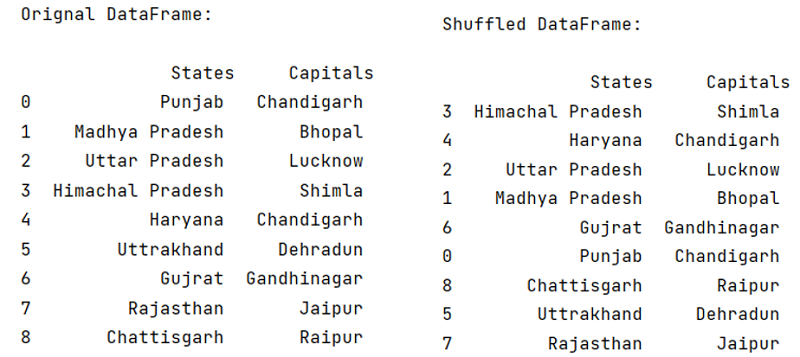# Python | Shuffle Pandas DataFrame Rows

In this tutorial, we will learn how to shuffle Pandas DataFrame rows with the help of example? By Pranit Sharma Last updated : April 12, 2023

Shuffling of rows means changing the sequence of rows randomly.

## Pandas Shuffle DataFrame Rows

To shuffle Pandas DataFrame rows (shuffle the order or rows), use sample() method. is an inbuilt method for shuffling sequences in python. Hence, in order to shuffle the rows in DataFrame, we will use DataFrame.sample() method. Shuffle method takes a sequence (list) as an input and it reorganize the order of that particular sequence.

### Syntax

```DataFrame.sample(
n=None,
frac=None,
replace=False,
weights=None,
random_state=None,
axis=None,
ignore_index=False
)

# or
DataFrame.sample(n=none, frac=none, axis= none)
```

Here,

• n: n is total number of rows to be shuffled.
• frac: frac is fraction of total instances needs to be returned, i.e., number of times shuffling needs to be done.
• axis: '0' or 'row' for rows and '1' or 'column' for columns.
Note

The sample() method does not work with immutable types of data types like strings.

## Python Code to Shuffle Pandas DataFrame Rows

```# Importing pandas package
import pandas as pd

# Creating a dictionary
d = {
'Haryana','Uttrakhand','Gujrat','Rajasthan','Chattisgarh'],
'Capitals':['Chandigarh','Bhopal','Lucknow','Shimla','Chandigarh',
}

# Creating a DataFrame
df = pd.DataFrame(d)

# Printing original DataFrame
print("\nOrignal DataFrame:\n\n",df)

# Shuffling the order of rows
# using the sample() method
df = df.sample(n=9)

# Printing the shuffled DataFrame
print("\nShuffled DataFrame:\n\n",df)
```

### Output

```
Orignal DataFrame:

States     Capitals
0            Punjab   Chandigarh
4           Haryana   Chandigarh
6            Gujrat  Gandhinagar
7         Rajasthan       Jaipur
8       Chattisgarh       Raipur

Shuffled DataFrame:

States     Capitals
6            Gujrat  Gandhinagar
4           Haryana   Chandigarh
7         Rajasthan       Jaipur
0            Punjab   Chandigarh
8       Chattisgarh       Raipur
```

### Output (Screenshot)